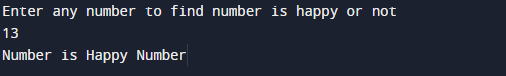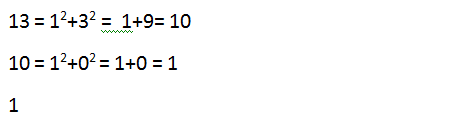C Program to print whether given Number is Happy or not

In this tutorial you will learn about the C Program to print whether given Number is Happy or not and its application with practical example.

In this tutorial, we will learn to create a C program that will find the given number is a happy or not  using C programming.

Prerequisites

Before starting with this tutorial we assume that you are best aware of the following C programming topics:

• C Operators.
• Loop statements.
• While and for Loop.
• Conditional statement.

What Is Happy Number ?

Happy number is a number which finally ends at 1 when it is replaced by the sum of the square of its digits repeatedly.

Program to print whether given Number is Happy or not ?

in this program we are going to find that the given number is happy or not ?.As we explained happy number is a number which finally ends at 1 when it is replaced by the sum of the square of its digits repeatedly.In this program we will find that given number is happy number or not using for loop.
Firstly we declare required header file and variable and also initiates required values in variable. Next we take value from user at run time and then after we will find that the given value is happy number or not.

OutputA number is said to be happy if it leads to 1 when we calculate the square of each digit present in number and them. if resulting sum is equal to 1 then the given number we can say it is a happy number.

example.

Let’s understand with an example:In our example we have taken 13 to find that this number is happy or not,we  first break 13 to get the sum of squares of its digits which gives us another number (10), we interchange 13 by 10 to continue this process until we get result as 1.So 13 a happy number.As we discuss  above  happy number is a number that will give 1 at the end on continues after a sequence of steps wherein each step number is replaced by the sum of squares of its digit.
In our example we can see that 13 is a happy number.

In this tutorial we have learn about the C Program to print whether given Number is Happy or not and its application with practical example. I hope you will like this tutorial.